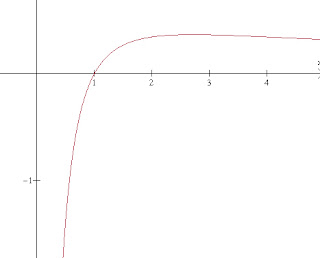## Saturday, 4 October 2008

### A Nice, but Tough, Problem

Click on images to see higher resolution

This one is for my old teaching friend, Al Harmon, who has asked and answered problems for me and from me for many years... This is a variation of a problem that was on the "Wild About Math" website, adjusted a little to make it more compatible to students at HS level..

If you take a number (let's use 200 for an example) and think of all the ways you could write it as a sum or positive integers... (100+100, or 2 + 105 + 93 or... 1+1+1+.....+1) these are called the partitions of the number. Now if you take all the integers in the partition and multiply them together, which one would give the largest product? Obviously 1+1+1...+1 will not amount to a very large product.... 100* 100 is lots larger, and 66* 67 * 67 is even larger...almost 3 (10 5),... which might make you think... aha...more small numbers is better... so 2100 will be great! ...Well it is better, its about 1.126 (1030 ) which is way larger than the others... but can we make it bigger???... What if we experiment a little with other values we try five, and find 5 40 = about 9 (10 27) .... big, but not as big as 2100 ...

well, what about 4 50... wait, that is exactly the same as 2100 by the exponent laws... so using all twos or all fours gives the same result... and fives yeilds less

Hmmmm..... We can't use all threes, but just for the heck of it, we try using two threes in place of three of the twos... what would 297 (32) give us...... aha... more.... just a little more, but more... about 1.46 (1030)... would more threes help??? would lots of threes help??? We could try using all threes and as many twos as would be left over at the end... 66 threes and 1 two would give us 6.18 (1031) more than any of the others. But Is THAT the largest... how can we convince ourselves that using threes is better than anything else?

Side trip to remember some ideas about logarithms.... remember that if a>b then ln(a) > ln(b) ... bigger numbers have bigger logs...so maximizing the product is the same as maximizing the log of the product.... and when we multiply numbers together, we are adding their logarithms to get the log of the product...[ln (bc)=lln (b) + ln(c)] ... now we can use that to think about how the log of the product changes...each time we use a number from the partition of the value, we add x to the sum, and ln(x) to the ln of the product.. so whatever number has the largest ratio of ln(x)/x will be the best choice to use.... If we graph the function (I used winplot, which is a wonderful FREE software.. ) we see the graph below.It seems to be highest somewhere between 2 and 3, and a calculus student should be able to figure out that happens when f'(x) = 0, and since the numerator of the derivative is 1- ln(x), we know that will happen when ln(x) = 1, or x=e..... but that is NOT an integer... but we do notice the slope is always positive to the left of x=e, and always negative to the right of x=e, so whichever one of the two adjacent integers is most efficient, will be the most efficient.. we find that ln(3)/3 > ln(2)/2 and our question is answered.

#### 1 comment:

Joshua Zucker said...

You don't need to bring e, or ln, into it.

All you need is that 3^2 > 2^3.

Then that shows that you want to have zero or one or two 2s and all the rest 3s (with the number of 2s determined by the value of your number mod 3, so with 200 you'll want to have one 2 and 66 3s).

Another fun question -- does e have to enter into this one?
Suppose that instead of partitioning n into integers with the largest product, you take n and choose a value of k, split n into k equal pieces, and multiply those pieces together. What value of k gives the largest result? In other words, for what integer k is (n/k)^k maximized?